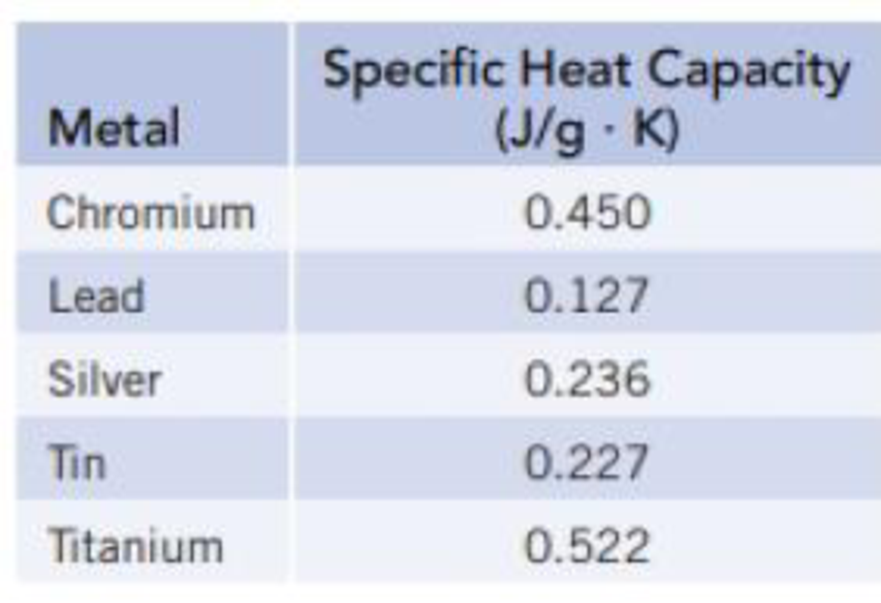Chapter 5, Problem 104SCQ

Chapter
Section
Textbook Problem

Prepare a graph of specific heat capacities for metals versus their atomic weights. Combine the data in Figure 5.4 and the values in the following table. What is the relationship between specific heat capacity and atomic weight? Use this relationship to predict the specific heat capacity of platinum. The specific heat capacity for platinum is given in the literature as 0.133 J/g · K. How good is the agreement between the predicted and actual values?Interpretation Introduction

Interpretation:

The graph comparing specific heat capacity with their atomic weights of metals and predict for the specific heat capacity of platinum has to be prepared.

Concept Introduction:

The specific heat capacity (C) is the energy transferred as heat that is required to raise the temperature of 1gram of substance by one kelvin.

Explanation

Given the table for specific heat capacity (C) and atomic weights of metals

 Metals specific heat capacity (C) in J/gK atomic weight Molar heat capacity in J/molK Al 0.897 26.98 24.2 Ti 0.522 47.867 24.9 Cr 0.450 51.99 23.3 Fe 0.449 55.84 25.1 Cu 0.385 63.54 24.5 Ag 0.236 107.86 25.4 Au 0.129 196.96 25.4 Pb 0.127 207.2 26.3 Pt 0.133 195.084 25.9

Table 1

Still sussing out bartleby?

Check out a sample textbook solution.

See a sample solution

The Solution to Your Study Problems

Bartleby provides explanations to thousands of textbook problems written by our experts, many with advanced degrees!

Get Started

What are the moral implications of using ergogenic aids?

Understanding Nutrition (MindTap Course List)

What kind of spectrum does a neon sign produce?

Horizons: Exploring the Universe (MindTap Course List)

Identify all chiral carbon atoms in citrate and isocitrate.

Chemistry for Today: General, Organic, and Biochemistry